Courses

# Theory & Procedure, Spectrometer (Prism) Class 12 Notes | EduRev

## JEE : Theory & Procedure, Spectrometer (Prism) Class 12 Notes | EduRev

The document Theory & Procedure, Spectrometer (Prism) Class 12 Notes | EduRev is a part of the JEE Course Physics Class 12.
All you need of JEE at this link: JEE

Our Objective:

(i) To study the angle of deviation (d) with angle of incidence (i) and to find the angle of minimum deviation (D) from i-d curve.

(ii) To find the refractive index of the material of the prism using A and D.

The Theory:

Prism

A prism is an optical element. It has polished flat surfaces that refract light. The traditional geometric shape of a prism has a triangular base and two rectangular sides. It is called triangular prism.

A prism can be made from materials like glass, plastic and fluorite. It can be used to split light into its components.

How a Prism Works

When light travels from one medium to another medium, it is refracted and enters the new medium at a different angle. The degree of bending of the light's path depends on the angle that the incident beam of light makes with the surface of the prism, and on the ratio between the refractive indices of the two media. This is called Snell's law.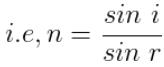where, n is the refractive index of the material of the prism.
i is the angle of incidence.
r is the angle of refraction.

The refractive index of many materials varies with the wavelength of the light used. This phenomenon is called dispersion. This causes light of different colors to be refracted differently and to leave the prism at different angles, creating an effect similar to a rainbow. This can be used to separate a beam of white light into its constituent spectrum of colors.

The relation between Refractive Index (n), Angle of Prism (A) and Angle of Minimum Deviation (D)

Consider the following triangular prism.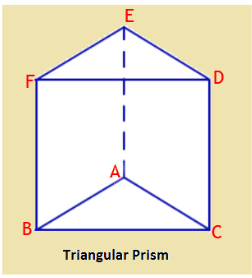The angle A between the two refracting surfaces ABFE and ACDE is called the angle of prism.

A ray of light suffers two refractions on passing through a prism. If KL be a monochromatic light falling on the side AB, it is refracted and travels along LM. It once again suffers refraction at M and emerges out along MN. The angle through which the emergent ray deviates from the direction of incident ray is called angle of deviation 'd'.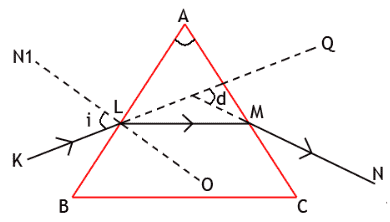As the angle of incidence is increased, angle of deviation 'd' decreases and reaches minimum value. If the angle of incidence is further increased, the angle of deviation is increased.

A graph is drawn between angle of incidence (i) and angle of deviation (d) by taking angle of incidence (i) along X-axis and angle of deviation (d) along Y-axis. It should be a curved graph.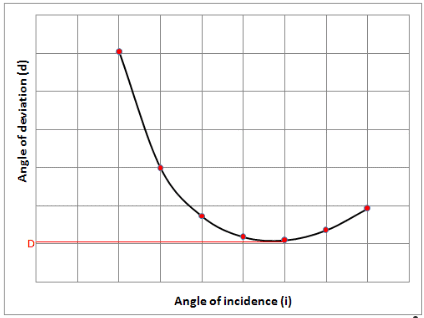The angle of minimum deviation is obtained from the graph. Let D be the angle of minimum deviation, then the refractive index (n) of the material of the prism is calculated using the formula,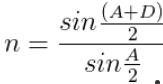Learning outcomes:

• Students understand the working of a prism.
• Students will be better able to do the experiment in a real laboratory by understanding the procedure.

Materials required:

• Glass prism
• Drawing board
• Paper
• Pins
• Scale
• Pencil
• Protractor

Real Lab Procedure:

• A paper is fixed on the drawing board placed on the table.
• Place the given glass prism on the center of the paper.
• Using the pencil, mark the outline ABC of the prism on the paper.
• Remove the prism, and using the scale and pencil, normal N1O is drawn to the face AB at the point L.
• Using the protractor, measure an angle 30° from the normal.
• Another line KL is drawn at L making the angle 30° (angle of incidence i) with the normal N1O.
• Two pins R1 and R2 are fixed on this line.
• The prism is replaced on the outline ABC.
• Viewing the pins from the face AC of the prism, two other pins R3 and R4 are fixed so that R1, R2, R3 and R4 are in a line.
• Remove the pins.
• A line NM is drawn to meet on the face AC through the marks of R3 and R4.
• The line LM is joined.
• The line KL is extended to get the LQ and NM is extended to get the line MP. These two lines meet at P.
• Using the protractor, measure the angle QPM. This is the angle of deviation d.
• Repeat the experiment for different values of angle of incidence (i) and the corresponding angle of deviations are measured.
• Draw a graph with angle of incidence (i) along the X-axis and angle of deviation (d) along the Y – axis.
• The angle of deviation corresponding to the lowest bend of the curve is the angle of minimum deviation (D).
• Angle of the prism A is directly measured from the outline of the prism using the protractor.
• Calculate the refractive index of the material of the prism using the formula,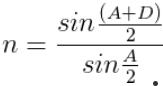Simulator Procedure (as performed through the Online Labs)

• Select the prism from the drop down list.
• Change the angle of incidence using the slider.
• For each angle of incidence (i), you will get corresponding angle of deviation (d), angle of refraction on the side AB (r1) angle of refraction on the side AC(r2) and angle of emergence (e).
• Click on the ‘Show/Hide protractor’ button to measure the angle using protractor.
• Click on the centre cross mark on the protractor to drag the protractor.
• Click on the rotate icon on the protractor to rotate the protractor.
• Click on the close icon on the protractor to close the protractor.
• You can use the embedded worksheet to enter the values.
• To see the graph, click on the graph icon in the worksheet.
• From the graph you will get minimum angle of deviation D.
• You can calculate the refractive index of the material of the prism using the equation,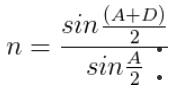• You can verify your result by clicking on the ‘Show result’ button.
• To redo the experiment, click the ‘Reset’ button.

Observations:

 Trial No. Angle of Incidence (i) Angle of Deviation (d) 1 30 2 35 3 40 4 45 5 50 6 55 7 60

Calculations:

• Angle of prism (A) = 60°.
• From graph, angle of minimum deviation D = ------------°
• Refractive index of the material of the prism,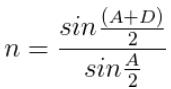Results:

• A graph showing the variation of angle of deviation with the angle of incidence is plotted.
• Angle of minimum deviation, D = --------°
• Refractive index of material of the prism, n = ------------
Offer running on EduRev: Apply code STAYHOME200 to get INR 200 off on our premium plan EduRev Infinity!

## Physics Class 12

204 videos|288 docs|125 tests

,

,

,

,

,

,

,

,

,

,

,

,

,

,

,

,

,

,

,

,

,

,

,

,

;